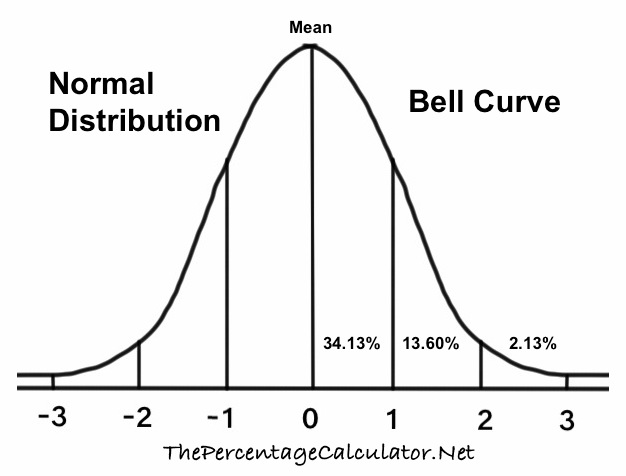Z Score -2We looked up the Z Score for -2 in our Normal Distribution Tables with Z Scores so you don't have to!

First, note that a Z Score of -2 means that your statistic is -2 standard deviation to the left of the mean on a bell curve. Here is a Bell Curve so you can visualize where -2 is on a bell curve.We used three different distribution tables, and we will give you the -2 Z Score probability, percentile, and explanations for all three.

Cumulative from mean
This gives you the probability of the area from the mean to your Z Score. The cumulative from-mean probability and percentile for a -2 Z score is displayed here:

0.477249868051821
= 47.7250%

Cumulative
This gives you the probability of the area below the Z Score. The cumulative probability and percentile for a -2 Z score is displayed here:

0.0227501319481792
= 2.2750%

Complementary cumulative
This gives you the probability of the area above the Z Score. The complementary cumulative probability and percentile for a -2 Z Score is displayed here:

0.977249868051821
= 97.7250%

Z Score Table Lookup
Here you can submit Z Scores between -3.999 and 3.999 for us to look up in our Normal Distribution Tables.

Z Score -1.999
Here is the next Z Score we looked up in our Normal Distribution Tables.

The percentiles on this page are rounded to the nearest 4 decimals.